Courses

# Aufbau Principle, Pauli's Principle and Hund's Principle Class 11 Notes | EduRev

## JEE : Aufbau Principle, Pauli's Principle and Hund's Principle Class 11 Notes | EduRev

The document Aufbau Principle, Pauli's Principle and Hund's Principle Class 11 Notes | EduRev is a part of the JEE Course Chemistry for JEE.
All you need of JEE at this link: JEE

The filling of electrons into the orbitals of different atoms take place on the basis of 3 principles :

Aufbau Principle:

The electrons are added progressively to Various orbitals in the order of increasing energy, starting with the orbital of lowest energy.

The energy of Orbitals: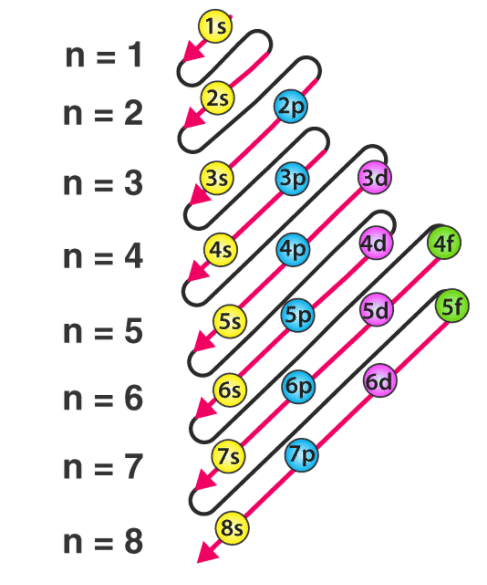(i) Hydrogen atom: In case of hydrogen atom, energy of orbital is mainly determined by principle quantum number.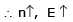For H atom

1s < 2s = 2p < 3s = 3p = 3d < 4s = 4p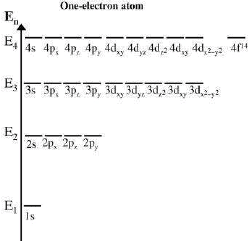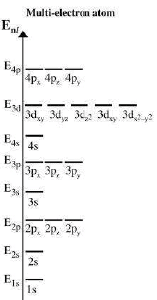(ii) Multi-electronic atoms: In case of multi-electronic atoms, energy of orbitals depends upon both n and l and hence energy of orbitals is compared on the basis of (n + l) rules generally.

⇒ n + l rule:

(iii) As the value (n + l) increases the total energy of orbitals also increases.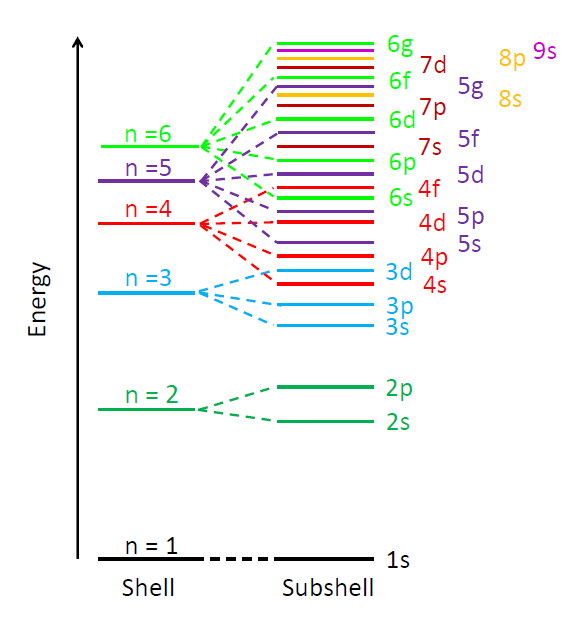Fig: energy levels.

(iv) If value of (n + l) is same or different orbital then the orbital with lower value of n, have lower energy.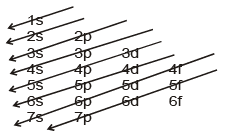1s < 2s < 2p < 3s < 3p < 4s < 3d < 4p < 5s < 4d < 5p < 6s < 4f < 5d < 6p < 7s

Pauli Exclusion Principle:
• The principle states that no two electrons in an atom can have same set of all 4 quantum no.
• Therefore spin quantum No. have only two values therefore it can also be commented that orbital can have maximum two electrons with opposite spin.
Hund's Rule of Maximum Multiplicity:

While filing the orbitals of same energy (i.e. orbitals of same subshell) pairing of electron start only when each orbital have got 1 electron that too with same spin or parallel spin.

Multiplicity is expressed as 2|s| + 1 where s represent total spin.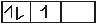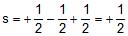2|s| + 1 = 2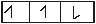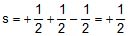, 2|s| + 1 = 2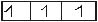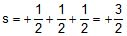, 2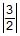+ 1 = 4 (It has maximum multiplicity)

Offer running on EduRev: Apply code STAYHOME200 to get INR 200 off on our premium plan EduRev Infinity!

## Chemistry for JEE

198 videos|447 docs|370 tests

,

,

,

,

,

,

,

,

,

,

,

,

,

,

,

,

,

,

,

,

,

,

,

,

;